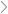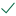Questions & AnswersInorganic ChemistryExperiments #8 &amp; #9 - Alkal inity (two-week lab) PRE-LAB...

QuestionAnswered step-by-step

# Experiments #8 & #9 - Alkal inity (two-week lab) PRE-LAB...

Experiments #8 & #9 - Alkal inity (two-week lab)

PRE-LAB ASSIGNMENT

1. You will start with ~0.5 grams of Na2CO3 and diluted it to a final volume of 100 mL. Calculate the approximate molarity of the solution?

1. You will take 20.0 mL of your "Standard Sodium Carbonate Solution" and diluted it up to 50 mL. Calculate the approximate volume of the 0.05 M (0.10 N) sulfuric acid solution sulfuric acid solution will be required to titrate 20.0 mL of your "Standard Sodium Carbonate Solution" to the first endpoint (EP1).

1. You will need to estimate how many mL of the 0.05 M (0.10 N) sulfuric acid solution sulfuric acid solution will be required to titrate 20.0 mL of your "Standard Sodium Carbonate Solution" to the first endpoint (EP2).Solved by verified expert10,000 step-by-step explanations